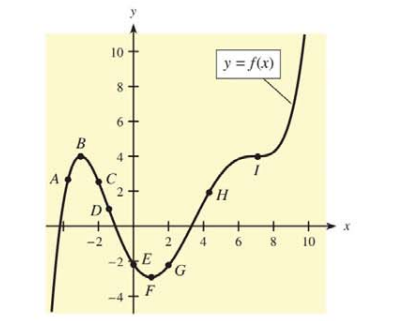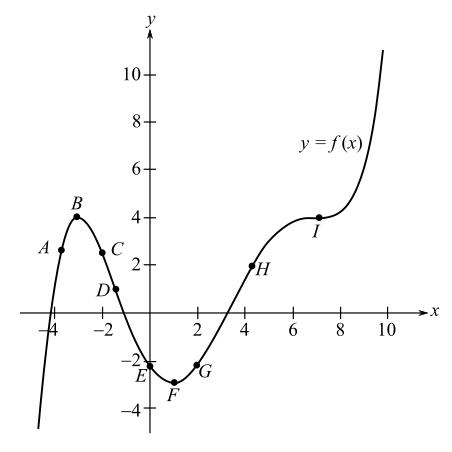Chapter 10.2, Problem 28E### Mathematical Applications for the ...

11th Edition
Ronald J. Harshbarger + 1 other
ISBN: 9781305108042

#### Solutions

Chapter
Section### Mathematical Applications for the ...

11th Edition
Ronald J. Harshbarger + 1 other
ISBN: 9781305108042
Textbook Problem

# In Problems 29 and 30, use the graph shown in the figure and identify points from A through I that satisfy the given conditions.(a) f ' ( x ) > 0  and  f " ( x ) < 0 (b) f ' ( x ) > 0  and  f " ( x ) > 0 (c) f ' ( x ) > 0  and  f " ( x ) < 0 (d) f ' ( x ) > 0  and  f " ( x ) = 0(a)

To determine

The point that satisfies f(x)>0 and f(x)<0 in the graph of the function f(x).Explanation

Given Information:

The provided graph of the function f(x) is

Explanation:

Consider the provided graph of the function f(x),

Since, the graph is of the function f(x).

Recall that if the function is concave up, then f(x)>0 and if it is concave down then f(x)<0.

And, is the point of inflection, where the concavity changes.

Recall that if the function is increasing, then f(x)>0 and if it is decreasing then f(x)<

(b)

To determine

The point that satisfies f(x)<0 and f(x)>0 in the graph of the function f(x).(c)

To determine

The point that satisfies f(x)=0 and f(x)<0 in the graph of the function f(x).(d)

To determine

The point that satisfies f(x)<0 and f(x)=0 in the graph of the function f(x).### Still sussing out bartleby?

Check out a sample textbook solution.

See a sample solution

#### The Solution to Your Study Problems

Bartleby provides explanations to thousands of textbook problems written by our experts, many with advanced degrees!

Get Started

#### Find more solutions based on key concepts